## Temperature and thermal expansion

11-29-99

Sections 13.1 - 13.6

We'll shift gears in the course now, moving from the physics of mechanical systems to thermal physics.

### Temperature scales

In the USA, the Fahrenheit temperature scale is used. Most of the rest of the world uses Celsius, and in science it is often most convenient to use the Kelvin scale.

The Celsius scale is based on the temperatures at which water freezes and boils. 0°C is the freezing point of water, and 100° C is the boiling point. Room temperature is about 20° C, a hot summer day might be 40° C, and a cold winter day would be around -20° C.

To convert between Fahrenheit and Celsius, use these equations:The two scales agree when the temperature is -40°. A change by 1.0° C is a change by 1.8° F.

The Kelvin scale has the same increments as the Celsius scale (100 degrees between the freezing and boiling points of water), but the zero is in a different place. The two scales are simply offset by 273.15 degrees. The zero of the Kelvin scale is absolute zero, which is the lowest possible temperature that a substance can be cooled to. Several physics formulas involving temperature only make sense when an absolute temperature (a temperature measured in Kelvin) is used, so the fact that the Kelvin scale is an absolute scale makes it very convenient to apply to scientific work.

### Measuring temperature

A device used to measure temperature is called a thermometer, and all thermometers exploit the fact that properties of a material depend on temperature. The pressure in a sealed bulb depends on temperature; the volume occupied by a liquid depends on temperature; the voltage generated across a junction of two different metals depends on temperature, and all these effects can be used in thermometers.

### Linear thermal expansion

The length of an object is one of the more obvious things that depends on temperature. When something is heated or cooled, its length changes by an amount proportional to the original length and the change in temperature: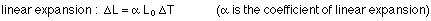The coefficient of linear expansion depends only on the material an object is made from.

If an object is heated or cooled and it is not free to expand or contract (it's tied down at both ends, in other words), the thermal stresses can be large enough to damage the object, or to damage whatever the object is constrained by. This is why bridges have expansion joints in them (check this out where the BU bridge meets Comm. Ave.). Even sidewalks are built accounting for thermal expansion.

Holes expand and contract the same way as the material around them.

### Example

This is similar to problem 12.20 in the text. Consider a 2 m long brass rod and a 1 m long aluminum rod. When the temperature is 22 °C, there is a gap of 1.0 x 10-3 m separating their ends. No expansion is possible at the other end of either rod. At what temperature will the two bars touch?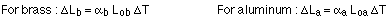The change in temperature is the same for both, the original length is known for both, and the coefficients of linear expansion can be found from Table 12.2 in the textbook.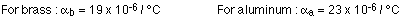Both rods will expand when heated. They will touch when the sum of the two length changes equals the initial width of the gap. Therefore: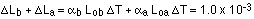So, the temperature change is: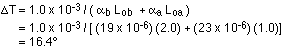If the original temperature was 22 °C, the final temperature is 38.4 °C.

### Thermal expansion : expanding holes

Consider a donut, a flat, two-dimensional donut, just to make things a little easier. The donut has a hole, with radius r, and an outer radius R. It has a width w which is simply w = R - r.

What happens when the donut is heated? It expands, but what happens to the hole? Does it get larger or smaller? If you apply the thermal expansion equation to all three lengths in this problem, do you get consistent results? The three lengths would change as follows: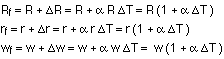The final width should also be equal to the difference between the outer and inner radii. This gives: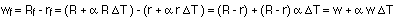This is exactly what we got by applying the linear thermal expansion equation to the width of the donut above. So, with something like a donut, an increase in temperature causes the width to increase, the outer radius to increase, and the inner radius to increase, with all dimensions obeying linear thermal expansion. The hole expands just as if it's made as the same material as the hole.

### Volume thermal expansion

When something changes temperature, it shrinks or expands in all three dimensions. In some cases (bridges and sidewalks, for example), it is just a change in one dimension that really matters. In other cases, such as for a mercury or alcohol-filled thermometer, it is the change in volume that is important. With fluid-filled containers, in general, it's how the volume of the fluid changes that's important. Often you can neglect any expansion or contraction of the container itself, because liquids generally have a substantially larger coefficient of thermal expansion than do solids. It's always a good idea to check in a given situation, however, comparing the two coefficients of thermal expansion for the liquid and solid involved.

The equation relating the volume change to a change in temperature has the same form as the linear expansion equation, and is given by: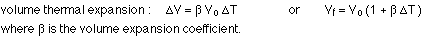The volume expansion coefficient is three times larger than the linear expansion coefficient.